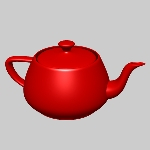Community Profile# Patrick Kalita

### MathWorks

Active since 2011

#### Statistics

All
•••••••••#### Content Feed

View by

Submitted

Scripts for Volume Visualization Videos
Source code files which go with the series of videos on volume visualization from Doug's Blog.Submitted

Waterfall Chart
This function shows incremental changes in a vector graphically in as a waterfall chart.

Plotting a 3D scalar field as a coloured 3D scatter graph
If you want to use <https://www.mathworks.com/help/techdoc/ref/scatter3.html |scatter3|>, you can pass it your |x|, |y|, and |z|...

7 years ago | 1

| accepted

creating 3D mesh for some points in space
<http://www.mathworks.com/help/matlab/ref/trimesh.html |trimesh|> is probably what you want to use. You can also use <http://www...

9 years ago | 2

Solved

Create a function handle that reverses the input arguments of another function handle
Given a function that takes two input arguments and returns one output, create another function handle that performs the same op...

9 years ago

Solved

Implement a counter
Write a function that returns a function that counts the number of times it is invoked. Example: >> h = counter; >> h() ...

9 years ago

how to setup a color matrix in surf(x,y,z,C) that has two components, one fixed, one related to data?
This approach certain has its limitations, but it's also pretty easy to implement: If you set the CData values on the border to ...

9 years ago | 0

| accepted

get rid of rugged edges plotted with trisurf
Try changing the figure's renderer to ZBuffer: set(gcf, 'Renderer', 'zbuffer');

9 years ago | 0

Matlab 3D view matrix
The matrix returned by |view| isn't terribly useful unless the axes' <http://www.mathworks.com/help/techdoc/visualize/f4-24991.h...

9 years ago | 0

Y- axis marking
Use the technique described here: <http://www.mathworks.com/support/solutions/en/data/1-16KCT/?solution=1-16KCT>

9 years ago | 0

Solved

Determine whether a vector is monotonically increasing
Return true if the elements of the input vector increase monotonically (i.e. each element is larger than the previous). Return f...

9 years ago

how to plot data points with different color in scatter plot?
You can use the fourth input argument to <http://www.mathworks.com/help/techdoc/ref/scatter.html |scatter|> to control the color...

9 years ago | 2

Solved

Solve the Sudoku Row
*Description* A simple yet tedious task occurs near the end of most Sudoku-solving algorithms, computerized or manual. The ta...

9 years ago

Solved

Parse string and identify specific string sequence in algebraic equation
Given a string S that defines an algebraic expression such as: S= 'X= A1 + A2*(Y1 + A3*Y3)*exp( A4*Y12 + Y1) ;' return a...

9 years ago

Solved

Pizza!
Given a circular pizza with radius _z_ and thickness _a_, return the pizza's volume. [ _z_ is first input argument.] Non-scor...

9 years ago

Colormap gradient on patch object together with a surface object
When the surface is added, MATLAB automatically changes the <http://www.mathworks.com/support/tech-notes/1200/1201.html#Section_...

9 years ago | 0

| accepted

how to allow user to get an arbitrary line profile from a contourplot
What you're asking to do isn't something that's going to have an off-the-shelf solution. _You need to build it yourself_. To...

9 years ago | 0

How to generate a date vactor of weekly intervals.
Start by defining the beginning of the vector using <http://www.mathworks.com/help/techdoc/ref/datenum.html |datenum|>: sta...

9 years ago | 0

| accepted

2D contourplots of a 3D function
I think you want to use <http://www.mathworks.com/help/techdoc/ref/slice.html |slice|>.

9 years ago | 0

| accepted

RGB-images on a 3D cube
This is an application for <http://www.mathworks.com/help/techdoc/visualize/f0-18164.html#f0-9250 texture mapping>. You can crea...

9 years ago | 4

| accepted

Simple 3D vector plotting
You're asking quite a lot there. Here are a few functions that will get you going in the right direction: * For drawing vecto...

9 years ago | 2

How to merge 3d plots of level planes into one plot (graph)
I'm not familiar with the |plotregion.m| function, but the first thing I would do is check to see if returns a graphics object h...

10 years ago | 0

3D surface plot in matlab

10 years ago | 0

changing the style in a loop with hold all...
This is the same basic idea as Kevin Holst's answer, but you don't need to set the default |LineStyleOrder| for all axes. You ca...

10 years ago | 2

export x and y data from a matlab plot into a matrix
Follow the steps outlined in this solution: <http://www.mathworks.com/support/solutions/en/data/1-A6PBV2/?solution=1-A6PBV2 How ...

10 years ago | 0

Rotate a figure created with the area function

10 years ago | 0

| accepted

Solved

Pull the variable y_correct from the Caller's Workspace
*Description* This highlights a very easy to use and high-scoring cheat that can be used on almost all Cody questions.

10 years ago

How to make MATLAB render 3D objects properly on close up?
Ah, okay. Here's what's going on. There are *three* axes camera properties that you need to worry about. You are currently only ...

10 years ago | 0

Plot a time series with the whole number not 10^(n)